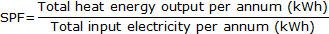Seasonal Performance Factor (SPF)

The Seasonal Performance Factor (SPF) only applies to heat pumps.

It is a measure of how efficiently your heat pump is operating. Put simply, the higher your SPF value the more energy efficient your system is.

SPF is a measure of the operating performance of an electric heat pump heating system over a year. It is the ratio of the heat delivered to the total electrical energy supplied over the year.

From 25 March 2016, your installer will calculate the SPF using the SCOP value from the MCS website. This number will be entered directly into the MCS database when the renewable technology is registered and will be the number used when calculating RHI payments. For more information on this, please see our Factsheet: ErP and the Domestic RHI.

The SPF is calculated the following way:This is to say, a heat pump with an SPF of 2.5 will on average deliver 2.5kWh of heat for every 1kWh of electricity it uses.

Your heat pump needs electricity to run (it powers the compressors). To keep your heat pump running as efficiently as possible (and electricity bills as low as possible) your property should be well insulated and have a low temperature heating system, such as underfloor heating or low temperature radiators. The lower the temperatures and the bigger the area of your radiators, the less electricity your heat pump will use and the higher its SPF will be.

Why is the SPF important?

The SPF is used to calculate the renewable portion of your heat pump’s heat output. The regulations require us to deduct the electricity used to run the heat pump from the heat it delivers. Only the renewable portion of the heat generated by your heat pump can be used when calculating payments.

To be eligible, all heat pumps must have a minimum SPF value of 2.5.

The SPF is a factor in payments calculations using the following formula:

 Payments = Estimated annual renewable heat load (total heat demand x (1– 1/SPF)) x tariff rate

Worked example

 Total heat demand 15,000kWh SPF 2.7 Example ground source heat pump tariff rate 19.86p/kWh SPF calculation (1-1/SPF), (1-1/2.7) = 0.63 Estimated annual renewable heat load (total demand x (1 - 1/SPF) 15,000kWh x 0.63 = 9,444kWh Total annual Domestic RHI payment (tariff x estimated annual heat load) £1,875.67 Quarterly Domestic RHI payment (total annual Domestic RHI payment / 4) £468.92

What if I’m metered for payment?

If you are metered for payment, your quarterly payments are based on actual meter readings where the electricity used is deducted from the heat output, so calculating the renewable heat generated.

Your SPF is still important. Your quarterly payments are based on your metered output BUT you will not be paid more than a set payment amount. There is a payment cap set so that you will not be paid more than you would have received if you were deemed. The payment cap takes into account your SPF and is based on your property’s estimated annual renewable heat load.

The SPF will be used to calculate the maximum amount you can be paid per year using the calculation in the following worked example:

 Using the worked example above: Annual Payment Cap = tariff x estimated annual renewable heat load:19.86p/kWh x 9,444kWh = £1,875.67